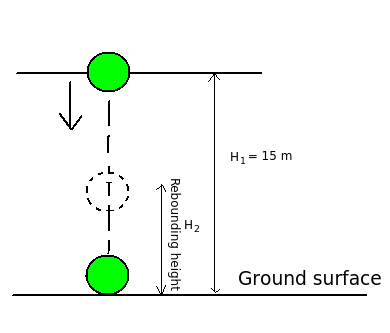# Rebounding height of ball dropped on horizontal surface

Q.  A ball is dropped on a horizontal surface from a height of 15 m. What will be the rebounding height of the ball after striking the surface? (coefficient of restitution = 0.8)
- Published on 18 Sep 15

a. 9.6 m
b. 6.5 m
c. 5.6 m
d. Insufficient data

#### Discussion

• zeeshan   -Posted on 22 Sep 20
formula is e=underroot(H2/H1)
• shilpi jain   -Posted on 24 Jun 16
thnks for the solution
• Sravanthi   -Posted on 16 Dec 15
Coefficient of restitution is defined as the ratio of impulse during restoration period to impulse during deformation period.

Given: Coefficient of restitution = 0.8, height of surface = 15 m

Formula: Coefficient of restitution (e) = √H1/H2

Solution:

When a ball is dropped from a height on a ground surface which has mass larger than mass of the ball, then the resulting impact is considered as the an impact with infinite mass. Due to impact, there is loss of kinetic energy and the law of conservation of momentum is not applicable.Coefficient of restitution (e) = √H1/H2

Substituting the given values,

0.8 = √15 / H2

H2 = 9.6 m

The rebounding height of the ball after striking the surface = 9.6 m

## ➨ Post your comment / Share knowledgeEnter the code shown above:

(Note: If you cannot read the numbers in the above image, reload the page to generate a new one.)